Selection prerequisites: One of the following combinations of point(s) and distance(s):

One point and one segment or distance value

Multiple points and one segment or distance value

One point and multiple segments and/or distance values

This Construct menu command constructs one or more circles centered at each selected point and with the radius determined by each selected segment or distance measurement.

This command duplicates the function of a noncollapsible compass, which allows you to set a length and then draw circles centered anywhere with that length as the radius.

 Selected Objects: Resulting Construction: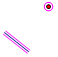One point, one segment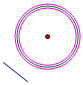One circle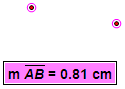Two points, one distance measurementTwo circles centered at the points with radius determined by the measurement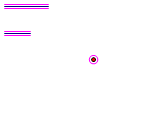One point, two segments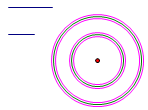Two circles centered at the point with radii determined by the segments